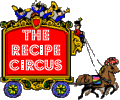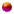Buy cookbooks at http://CookbooksPlus.commember logonabout the Circussearch for recipesprint this recipemimi's cyber kitchenfree registrationmember pageswhat's newemail this recipediscussion boardsEmail to Karen

Recipe Categories:

# Meat .. Points

WW Post

### List of Ingredients

Beef:
(Source: Ohiobeef.com,Nutritiondata.com and txbeef.org)
(fat trimmed to 1/4 inch)
Cut, oz (calories/fat)=points
No fiber in beef
4-oz raw = 3-oz cooked

Roasts:

7 Bone Pot Roast, 3-oz (293/22.6)= 6 points
Brisket, 3-oz (309.4/22.6)= 8 points
Boneless Rump Roast, 3-oz (170/7)= 4 points
Boneless Shoulder Roast, 3-oz (205/8)= 5 points
Boneless Round Roast, 3-oz (220/12)= 5.5 points
Bottom Round Roast, 3-oz (205/8)= 4 points
Chuck Arm Roast, 3-oz (282/20.2)= 7.5 points
Chuck Blade Roast, 3-oz (290/21)= 7.5 points
Chuck Eye Roast, 3-oz (282/23.2)= 7.5 points
Corned Beef, 3-oz (213/16.1)= 6 points
Cross Rib Pot Roast,3-oz (282/20)=7.5points (other names)*
*-Boston Cut, 3-oz (282/20)= 7.5 points
*-English Cut 3-oz (282/20)= 7.5 points
*-Thick Rib Roast 2-oz (282/20)= 7.5 points

Eye Round Roast, 3-oz (170/7)= 4 points
Pikes Peak Roast, 3-oz (170/7)= 4 points
Prime Rib Roast, 3-oz (290/23) = 8 points
Rib Roast, large end 3-oz (300/24)= 8 points
Rib Roast, short end 3-oz (280/28)= 7.5 points
Ribeye Roast, 3-oz (280/21)= 7 points
Tenderloin Roast, 3-oz (180/8.5)= 4 points
Tri-Tip Roast, 3=oz (177/8.2)= 4.5 points

Steaks:
(fat trimmed to 1/4 inch)
cut, oz (calories/fat)= points
4-oz raw = 3-oz cooked

Boneless Top Blade Steak, 3-oz (293/22)= 7 points*
*- Flat Iron Steak, 3-oz (293/22)= 7 points
Chuck Eye Steak, 3-oz (282/23.2)= 7.5 points
Underblade steak, 3-oz (282/23.2)= 7.5 pts
Chuck Shoulder Steak, 3-oz (293/22.6)= 7.5 points
Eye Of Round Steak, 3-oz 150/5)= 3.5 points (other names)*
*-Ball Tip Steak, 3-oz (150/5)= 3.5 points
*-Beef Round Steak, 3-oz (150/5)= 3.5 points
*-Breakfast Steak, 3-oz (150/5)= 3.5 points
*-Knuckle Steak, 3-oz (150/5)= 3.5 points
*-London Broil, 3-oz (150/5)= 3.5 points

Filet Mignon, 3-oz (175/8)= 4.5 points (other names)*
*-Tenderloin Steak, 3-oz (175/8)= 4.5 points
*-Medallion Steak 3-oz (175/8)= 4.5 points

Flank Steak, 3-oz (192/10.6)= 5 points
Flank Stek Rolls, 3-oz (192/10.6)= 5 points
Rib-Eye Steak, 3-oz (195/10)= 5 points (other name)*
*-Beauty Steak, 3-oz (195/10)= 5 points
*-Delmonico Steak, 3-oz (195/10)= 5 points
*-Market Steak, 3oz (195/10)= 5 points
*-Spencer Steak, 3-oz (195/10)= 5 points

Poterhouse Steak, 3-oz (260/18.8)= 7 points
Round Steak, 3-oz (170/7)= 4 points
Round Tip Steak, 3-oz (183.6/25.5)= 6 points
Sirloin Steak, 3-oz (180/7)= 4.5 points
Sirloin Tips, 3-oz (185/8)= 4.5 points
Skirt Steak, 3-oz (190/9)= 5 points * (other names)
*-Fajita Meat, 3-oz (190/9)= 5 points
*-Inside or Outside Skirt Steak, 3-oz (190/9)= 5 points
*-Roumanian Steak 3-oz (190/9)= 5 points

T-Bone Steak, 3-oz (253/18)= 7 points
Top Sirloin Steak, 3-oz (180/7)= 4.5 points (other names)*
*-Ambassador Steak, 3-oz (180/7)= 4.5 points
*-Club Steak,3-oz (180/7)= 4.5 points
*-Hotel Steak, 3-oz (180/7)= 4.5 points
*-KC Strip, 3-oz (180/7)=4.5 points
*-New York Strip Steak, 3-oz (180/7)= 4.5 points
*-Shell Steak, 3-oz (180/7)= 4.5 points
*-Strip Steak, 3-oz (180/7)= 4.5 points

Tri Tip Steak, 3-oz (177/8.2)= 4.5 points

Ribs:
cut, oz (calories/fat)= points
all weight is cut off the one

Back Ribs, 3-oz (310/22.2)= 8 points
Barbecue Ribs 3-oz (310/22.2)= 8 points
Short Ribs, 3-oz (293/22.2)= 7.5 points
Chuck Short Ribs, 3-oz (293/22.2)= 7.5 points
English Short Ribs,3-oz (292/22.2)= 7.5 points
Kosher Ribs, (292/22.2)= 7.5 points

GROUND Beef:
cooked (calories/fat)= pts
4-oz raw = 3-oz cooked
(90% lean or leaner 3 1/2-oz raw = 3-oz cooked)

beef 75/25, chuck 80/20, sirloin 85/15, round 90/10 or higher

Beef, 75% lean 3-oz (235/15) = 6 pts
Beef, 75% lean 1-lb (1253/80 = 31.5 pts
Beef, 80% lean 3-oz (231/15) = 6 pts
Beef, 80% lean 1-lb (1232/80) = 31.5 pts
Beef, 85% lean 3-oz (218/13) = 5.5 pts
Beef, 85% lean 1-lb (1162/69) = 29 pts
Beef, 90% lean 3-oz (196/10) = 5 pts
Beef, 90% lean 1-lb (1045/53) = 25.5 pts
Beef, 95% lean 3-oz (164/6) = 4 pts
Beef, 95% lean 1-lb (874/31)= 20 pts
Beef, 96% lean 3-oz (140/4.5)= 3.5 pts
Beef, 96% lean 1-lb (746.6/25.5)= 17 pts
Beef, Healthy Choice 97% lean 3-oz (131/4)= 3 pts
Beef, Healthy Choice 97% lean 1-lb (523/16.1)= 12 pts
Beef, 75% lean (240/20)= 6.5 pts
Chuck, 83% lean (240/16.7)= 6 pts
Sirloin, 90% lean (199/12.3)= 5 pts

Other Cuts:

Beef Heart, 3-oz (133/4)= 3 points
Beef Liver, 3-oz (200/9)= 5 points
Beef Soup Bones, 3-oz (171/5)= 4 points
Beef Tongue, 3-oz (210/14)= 5.5 points
Beef Tongue, canned, pickled, 3-oz (230/17)= 6.5 points
Mountain Oysters, 1 (143/5)= 3 points
Stew Meat, Chuck 3-oz (280/20)= 7.5 points
Stew meat, lean (180/7)= 4.5 points
Cube Steak, 3-oz (155/7)= 4 points
Beef Jerky, 1-oz (90/2)= 2 points
Hot Dogs, beef, 1 dog, (170/14)= 5 points

**********************
Pork:
(Source: Farmland.com and Nutritiondata.com)
(fat trimmed, lean)
(4-oz raw = 3-oz cooked)
cut, oz (calories/fat)=points

Hams:

95% lean Ham, 3-oz (112/4)= 3 points
Bone-in Smoked Picnic, 4-oz (250/20.2)= 7 points
Bone-in Shank, 3-oz (183/8.9)= 4.5 points
Boneless Ham, 3-oz (175/7)= 4 points
Half Ham, 3-oz (175/7)= 4 points
Honey Baked Ham Co. 3-oz (170/9)= 4.5 points

Roasts:

Bone-in Blade Roast, 3-oz (197/12)= 5 points
Bone-in Sirloin Roast, 3-oz (164/5.7)= 4 points
Boneless Blade Roast, 3-oz (197/12)= 5 points
Boneless Butt Roast, 3-oz (197/12)= 5 points
Boneless Center Loin, 3-oz (165/6.1)= 4 points
Boneless Loin Roast, 3-oz (165/5.9)= 4 points
Boneless Sirloin Roast, 3-oz (164/5.7)= 4 points
Center Loin Roast, 3-oz (165/6.1)= 4 points
Center Rib Roast, 3-oz (182/8.6)= 4.5 points
Picnic Roast, 3-oz (145/6)= 4 points
Tenderloin Roast, 3-oz (139/4.1)= 3.5 points
Top Loin Roast, 3-oz (165/6)= 4 points

Chops:

Bone-in Loin, 1 chop 3-oz (191/11)= 5 points
Boneless Center Loin, 3-oz (150/6.5)= 3.5 points
Boneless Sirloin Chop, 3-oz (171/6.9)= 4 points
Butterfly Chop, 3-oz (150/6.5)= 4 points
Center Loin Chop, 3-oz (150/6.5)= 4 points
Center Rib Chop, 3-oz (190/9)= 5 points
Loin Chop, 3-oz (149/6)= 3.5 points
Rib Chop, 3-oz (175/8)= 4.5 points

Steaks:

Blade Boston, 3-oz (232/13)= 6 points
Center Cut Steak, 3-oz (130/4.5)= 3.5 points
Sirloin Cutlet Steak, 3-oz (130/4)= 3 points
Cube Steak, 2-oz (120/5)= 3 points

Ribs:

Babyback, 3-oz (about 4 ribs)(251/18.3)= 7 points
Country-style Ribs, 3-oz (281/16.8)= 7 points
Country-style Ribs, boneless, 3-oz (251.6/18.3)= 7 points
Spare ribs, 3-oz (337/26)= 9 points

Other Cuts:

Bacon, 1 slice (43/3)= 1 point
Bologna, 1 slice (69/6) 2 points
Bratwurst, Johnsonville, 1 link (290/25)= 8 points
Brotwurst, 1 link (260/19)= 7 points
Canadian Bacon, 2 slices (89/4)= 2 points
Chitterlings 3-oz (201/17)= 5.5 points
Ground Pork, 3-oz (252/18)= 7 points
Heart, 3-oz (215/7)= 5 points
Hot Dog, 1 link (204/7)= 5 points
Pepperoni, 15 slices (135/12)= 4 points
Pickled Pigs feet, 1-oz (57/5)= 2 points
Sausage, fresh, regular grind, 2 links (163/14)= 4.5 points
Sausage, 1 pattie, (190/6)= 4.5 points
Sausage Italian, 1 link (286/23)= 7.5 points
Sausage Polish, 1 link (326/29)= 8.5 points
Spam, 2-oz 1/2 thick (174/150= 5 points
Stew meat, lean 3-oz (120/5)= 3 points

**********************

LAMB:
Cut, oz, calories/fat)= points
4-oz raw or 3-oz cooked

Roasts:

Boneless Shoulder, 3-oz(175/8)= 4.5 pts
Center Leg, 3-oz (178/8)= 4 pts
Crown Roast, 3-oz (180/10)= 4.5 pts
Leg of lamb, 3-oz 162/7)= 4 pts
Loin Roast, 3-oz (162/7)= 4 pts
Rib Raost, 3-oz (250/12)= 6 pts
Shank Half, 3-oz (207/11)= 5 pts
Sirloin Roast, 3-oz (165/8)= 4 pts

Steaks:

Leg Center Slice, 3-oz (197/12)= 5 pts
Sirloin, 3-oz (165/7)= 4 pts
Blade Steak, 3-oz (197/12)= 5 pts

Chops:

Arm Chop, 3-oz (197/8)= 5 pts
Bone-in Chop, (185/8)= 4.5 pts
Boneless Blade chop, 3-oz (160/6.6)= 4 pts
French Rib Chop, 3-oz (197/80= 5 pts
Loin Chop, 3-oz (185/8)= 4.5 pts
Rib Chop, 3-oz 178/10)= 4.5 pts
Shoulder Blade Chop, 3-oz (230/10)= 5 pts
Sirloin Chop, 3-oz (165/7)= 4 pts

Ribs:

Boneless Ribs, 3-oz (197/8)= 5 pts
SpareRibs, 3-oz (304/14)= 7.5 pts

Other Cuts;

Cube Steak, 3-oz (197/80= 5 pts
Cube for Kabobs, (180/10)= 4.5 pts
Stew Meat, 3-oz (195/10)= 5 pts
Grounds, 3-zo (241/16.7)= 6.5 pts

**********************

POULTRY:
3-oz cooked

Chicken, boneless skinless breast 3-oz(125/1.4) = 3 pts
Chicken, drumstick, no skin (130/4.0) = 3 pts
Chicken, thigh, no skin (150/7.0) = 4 pts
Chicken, wing, no skin (150/6.0) = 4 pts
Turkey, bacon (louis rich) (33/2) = 1 pt
Turkey, 3-oz breast meat (180/3) = 4 pts
Turkey, wing (200/3) = 4 pts
Turkey, drumstick (170/4) = 4 pts
Turkey, Leg roasted-smoked from parks (151/7.5) = 3.5pts
Turkey, 3-oz thigh (160/5) = 4 pts
Cornish Hen (85/2.5) = 2 pts
Duck (w/out skin) 3-oz (90/5) = 2 pts

Ground Poultry:
4-oz raw = 3-oz cooked
(ground turkey breasts only 3 1/2-oz raw = 3-oz cooked)

Chicken, regular grind 3-oz (218/13) = 5.5 pts
Chicken, breast only grind 3-oz (140/3) = 3 pts
Turkey, regular grind 3-oz (200/11) = 5 pts
Turkey, breast only grind 3-oz (115/1) = 2 pts

Other Cuts:

Hotdog, Chicken 1 link (116/9)= 3 pts
Hotdog, Turkey 1 link (102/8)= 2 pts

**********************

GAME And Unusual Meats: (source: www.Nutritiondata.com & caloriecount.com) 4-oz raw or 3-oz cooked

Alligator, cooked (3-oz) (110/2)= 3 pts
Armadillo, raw (3oz) (150/3.7) = 3 pts
Beaver, roasted (3oz) (210/4.3) = 5 pts
Buffalo/Bison:
(souces: thebuffaloshop.com)
Ground, 85% lean 3-oz (202/13) = 5 pts
Ground, 97% lean 3-oz (131/4) = 3 pts
Chuck Shoulder Clod, 3-oz (164/5) = 4 pts
Ribeye, 3-oz (150/5) = 3.5 pts
Top Round, 3-oz (148/4) = 3.5 pts
Top Sirloin, 3-oz (145/5) = 3.5 pts

Boar 3-oz (139/3.7) = 3 pts
Caribou 3-oz(144/3.7) = 3 pts
Crocodile, 4-oz raw (142/3.4)= 3 pts
Deer/Venison:
Chuck roast 3-oz (135/2.5) = 3 pts
Ground cooked 3-oz(159/7) = 4 pts
Round steak 3-oz(129/2) = 3 pts
Tenderloin roast 3-oz(127/2)= 3 pts
Top Round, 3-oz (148/4) = 3.5 pts
Sausage, 3-oz (195/10) = 5 pts
Sticks, 1-oz (100/5) = 2.5 points

Duck 3-oz (w/o skin) (90/5) = 2 pts
Elk 3-oz(126/1.7) = 3 pts
Frogs Legs, fried in flour (3oz) (250/3.2) = 5 pts
Goat, 3-oz (124/2.6) = 3 pts
Goose, roast, meat only 3.5 oz (275/4.5) = 6 pts
Guinea Fowl, roast, lean only 3.5 oz (145/2.5)= 3 pts
Moose, 3-oz(152/2)= 3 pts
Ostrich, 3-oz (97/2/)= 2.5 pts
Pheasant, breast, roasted 3 oz (120/3.5) = 3 pts
Quail, breast, roasted 3 oz (105/3.) = 2 pts
Rabbit 3-oz(154/6.2) = 4 pts
Snake, Rattle, 4-oz raw (182/2.3) = 4 pts
Squirrel(3oz) (180/3.2) = 4 pts
Turkey, Wild, breast 3-oz (163/1.5) = 3.5 pts

### Recipe